Next: 5.3 The Effect of Phonon-Phonon Scattering Up: 5.2 The Long-Wavelength Problem Previous: 5.2 The Long-Wavelength Problem   Contents

## 5.2.1 Scattering Rates

In this work, the thermal conductivity in the silicon nanowires is calculated using the phonon lifetime approximation in the phononic Boltzmann transport equation (Eq. 2.72). For the calculation of the relaxation times, we adopt the bulk formalism for Umklapp scattering [118,119,57]: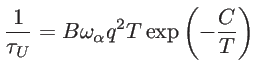(5.1)

where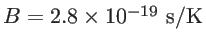and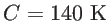. For boundary scattering we use: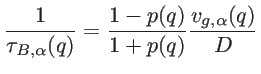(5.2)

where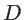is nanowire diameter and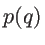is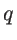-dependent specularity parameter given by [61,60,112]: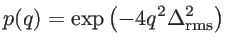(5.3)

where we used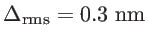for the root-mean-square of the roughness amplitude. The specularity parameter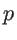taking values from 0 to 1, is determined by the details of the surface and surface roughness. For a smooth surface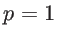, and the phonon-boundary scattering is fully specular. For a very rough surface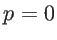, which results in fully diffusive boundary scattering. Recently, studies showed that experimental results for boundary scattering in nanowires of diameters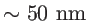are only explained if an almost fully diffusive boundary is assumed [131,132]. The overall relaxation rate including all scattering mechanisms is computed using Matthiessen's rule.

When considering the classical description of the energy distribution of phonons, in the Debye approximation, the heat conductivity of acoustic modes is given by [61,121]: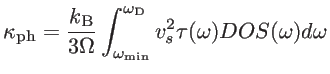(5.4)

where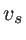is the corresponding sound velocity,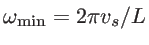is the minimum allowed phonon frequency which is determined by the longest allowed wavelength at a given channel length, and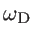is the Debye frequency. As the bulk density-of-states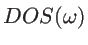is proportional to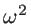, the Umklapp-limited thermal conductivity in 3D (with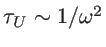as shown in Eq. 5.1) is bounded, even when the contribution of the long MFP phonons (as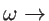) is included:(5.5)

since the second term of Eq. 5.5 goes to zero as the length increases.

In the case of 1D structures, on the other hand, the DOS is finite for low frequencies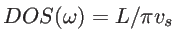, which does not allow the cancellation of theterm. Therefore, the Umklapp-limited thermal conductivity of a 1D system is given by: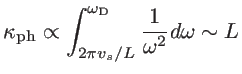(5.6)

indicating a linear divergence with the channel length. Physically, this means that the lowest wavevector that contributes to thermal conductivity is determined by the length of the channel. For an infinite channel, longer and longer wavevectors of finite DOS are involved, which causes divergence in the thermal conductivity, in contrast to bulk. Several works in the literature attempt to add corrections that would bound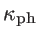as the channel length increases [120,126], although largecould still be possible in 1D. Some authors still use the bulk dispersion even for ultra-narrow channels [112,133,134], others include a constant specularity parameter for surface roughness, that adds a constant term in the total scattering rate, and removes the singularity for[123,135,101,136]. A different approach was proposed recently by Mingo et al., where an additional rate of a second order 3-phonon scattering mechanism without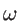-dependence was introduced for thermal transport in carbon nanotubes as :(5.7)

Here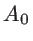is a frequency independent constant. This is just an order of magnitude approximation which removes the singularity for low frequency phonons, although very high thermal conductivities are still achieved. A study of the exact solution of the phonon BTE in carbon nanotubes showed a saturation inas the length increased to the millimeter range , which could prove the suitability of using Eq. 5.7.Next: 5.3 The Effect of Phonon-Phonon Scattering Up: 5.2 The Long-Wavelength Problem Previous: 5.2 The Long-Wavelength Problem   Contents
H. Karamitaheri: Thermal and Thermoelectric Properties of Nanostructures